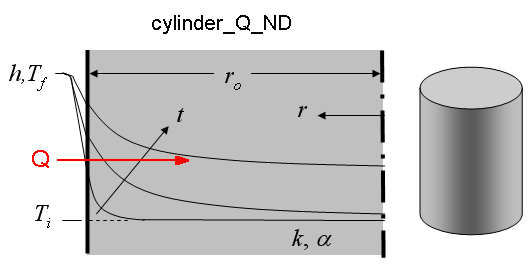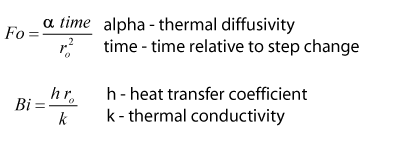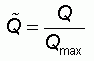Contents - Index

Cylinder_Q_NDFunction cylinder_Q_ND(Fo, Bi) returns the non-dimensional heat transfer within a long circular cylinder with the outside edge exposed to convection (r=r_o). The supplied values, Fo, Bi represent the Fourier number and Biot number respectively.  A separation of variable technique is used as described in Section 3.5.2 of Nellis and Klein.

Inputs:Output:Example:
Fo=0.23
Bi=1.02
Q_hat=cylinder_Q_ND(Fo, Bi)

{Solution: Q_hat=0.319}

Transient Conduction Index